Select Page

### Phi (ϕ) and PI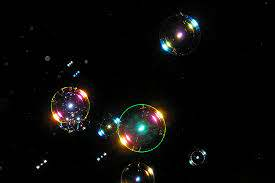After two years, we are finally getting to Spherical Time and the time bubble that cradles our existence.

Where do we get the simplest explanation for the sacred cubit to be 27.5”? We have to go back to our discussion in chapter VII of this series about the Phi(ϕ) angle, 137.50֯. Quite simply, 27.5 is (2 x 137.50֯) or 275.  Given the significance of the Phi(ϕ) field to the creation of physicality we need look no further. Why 2 times though? Because each inch of the cubit also had to align with the elements of the field of the expanded YHVH (יהוה) that likewise totals 275.  This number 2 is hinted at in the first letter of the Torah (ב) of numerical value 2. As previously explained, when spelled out the letter (בית) has a numerical value of 412, which is (3 x 137.50֯), as in the 3 components of the letter Bet (ב), 3 turns of the Phi(ϕ) clock within the circle of Creation. For anyone that has not been following this series from its inception, you can find the entire work here.

If the Torah’s first letter, Bet (ב) were 2֯ on a circle, the corresponding remainder of that circle, would be 358, the numerical value of Moshiach.

The second (complementary) part of the Phi(ϕ) angle, 137.50֯ is 222.50֯, as they add up to the 360֯ circle. So please note that the relationship of the two Triplets of the first word of the Torah breaks down to (203/913) = .222 and (710/913) = .778, allusions to the Phi(ϕ) angle and the number and year 5778 of Phi18 respectively. As we prepare to unwind the structure of our spherical time bubble, it is significant to note that the square root of 18 is 4.242640….

Most of us know that the 18 is the sum of the double letters Tet (טט) in the name Metatron or numerical value 314. What it would be odd for us to know is that the double letters Tet (טט) are found a single time in the Torah, and that all the doubled letters from Tet (טט) to Tav(תת) are collectively found 1729 times, as in the Taxicab number 1729, which is (93 + 103) and/or (13 + 123) and the exact number of occurrences of the word value 26 in the Torah, which is both the number of elements in a cube (26) and the value (26) of the YHVH (יהוה), not to mention the sum of the digits in (13 + 123 = 93 + 103). By the way, the sum of the digits in 1729 is 19, the back of the YHVH (יוד־הי־ויו־הי). This is showing us higher dimensional mathematics at work in the design of the Torah, fields intersecting above, filtering every letter and number through the Names of G-d fields and the cubic structure of Zeir Anpin before projecting onto the Torah and our world.  The average of all occurrences of all 22 doubled letters within words is 107.7273, as in the sum of the integers from 1 – 107 that equals Phi18 or 5778.

The most famous doubled Tet(טט) is not found in the Torah, but in the name of the archangel Metatron (מטטרון) of numerical value 314, connecting the 18 with PI.

The square root of 18 reminds us of Tesla’s 3-6-9, in that the √3 = 1.7320… the √6 = 2.449… and √9 = 3. While 173 is the small gematria of the 42-Letter Name and 2449 is the first year after the receipt of the Torah and √3 x √6 = 4.242640, the result of √3 x √6 x √9 = 12.73, or the Universal Harmonic (1.273).

As we explained earlier, this angle 137.50֯ is the Phi(ϕ) angle and is the reason for nature’s efficiency in terms of growth.  If a flower, tree, seashell, galaxy or anything branches outward, one turn of either 137.50֯  or 222.50֯ at a time, as it spirals outward from a central point, it will achieve the most efficient use of time and space possible, thus exerting the least amount of energy necessary for the maximum growth and preservation and propagation of life.

If you recall, the Alef-bet split alternating into odds-evens ordinally interacts with the Alef-bet values split according to the Phi(ϕ) proportion when we apply them to a 360֯ circle.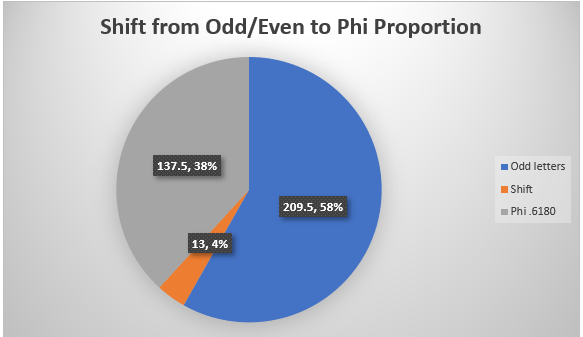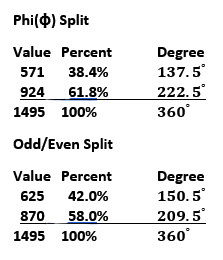Both these splits are primordial to the physical universe. Both existed with the interactions of the fields before physicality was produced. Both these proportions, Phi(ϕ) and 42/58 existed before the 22 Alef-bet fields, both predicated on even higher-level direction and purpose.

What is also primordial but not mathematically logical in any sense, other than in higher dimensional mathematics, is why the greater Phi(ϕ) angle, 222.50֯ less the smaller odd/even angle, 150.50֯, is equal to 72֯, which was also the proportion of the smaller odd/even split to the larger one: (625/870 = 72). Again, it was not that the Alef-bet just happened to divide up this way, but that it was guided to and created so that it would by the interactions of the Names fields prior to the creation of the 22 Alef-bet fields. This brings up another core relationship between 42-58-72 that Moshe and I have been discussing, which we will explain shortly, meanwhile…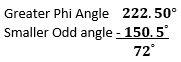The shift of 54 units, or 13֯ caused a 72֯ relationship to appear.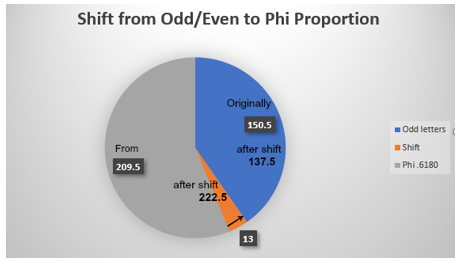Conversely, the lesser Phi(ϕ) angle 137.50֯ plus the odd angle 150.50֯ equals 288֯, which is (360֯ less 72֯).  With the entire Torah is based on the number 5 and with Phi(ϕ) mathematically based on  is it more than curious that 72 is 1/5 of 360, one-fifth of the circle. In Kabbalah 288 is representative of the 288 primordial Holy Sparks that Man was charged with recuperating in order to bring about the final redemption.Then when we used the two larger angles, the larger Even angle and the Greater Phi(ϕ) angle 222.50֯ we got (209.50֯  + 222.50֯ ) = 432֯ = (360֯  + 72֯) and the frequency 432 Hz, derivable from the Torah’s first and last word as we have just seen.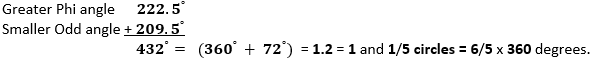This last relationship of 6/5 X 360֯ is interesting because a circle is dependent on Pi (3.14159…) for its formation utilizing the formula:This is not causational and may just be coincidental, though it may also be a simple way of showing us that the odd/even ordinal splitting of the Alef-bet also takes into account the relationship between the fields of Phi and Pi.

# The Seed of Spherical Time

Time begins at the intersection of the Pi and Phi fields. Every time Phi or the Phi(φ) angle wraps around the circle it deposits another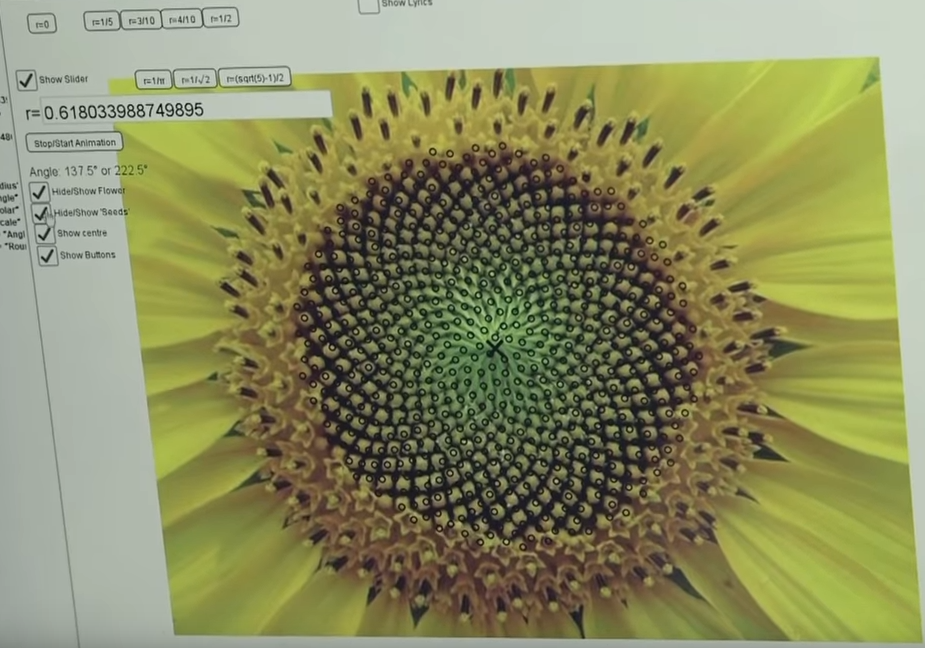unique point on the circle and does so infinitely. The natural spinning of Phi(φ) creates infinite radii of the same length. These are infinite pathways, infinite potentialities, infinite possibilities within a given circle. Given a set radius, those infinite point potentials form a circle. We could say that Phi(φ) in motion creates the circle that is defined by Pi. If that motion is in 2 perpendicular directions, we create a 3-dimensional sphere.

This is the time bubble. At a given radius, there are infinite possibilities. Time is the radius. At a given moment in time there exist an infinite set of possibilities emanating from the point of origin (Creation). That radius is proportionate to Phi(φ).

This is spherical time. A time bubble happens when there is a limit to the radius, which we call the event horizon.  More on this shortly.

# The Cubit

In the last part of this chapter we showed that the cubit (27.5”) = (12”)2/2φ2, which means that 1 cubit = (1’)2/2φ2, and that (27.5”) = (12”)2/2φ2 also equals 722, so 1 cubit = 722. This in turn means that 72/27.5 = φ2.

We see that the sacred cubit is based on 2 x Phi(ϕ) angle 137.50֯ = 27.5 and that it is also based on 722 while 72 is 1/5 of a circle 360֯. We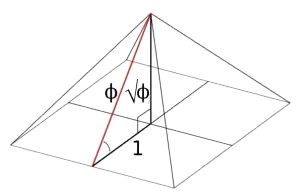use the cubit to make solid construction, but it is just a measure, the way a light-year or a mile is just a measure. It is the result of a specific confluence of fields, making it more than a number. Is it a measure of time? That is the construct of time, not the arbitrary 60 seconds in a minute 60 minutes in and hour, etc. A year is based on an orbit, an orbit is based on Pi. The orbit is based on a defined mechanical structure of our solar system of squares and circles and spheres, not on chance.  It is based on Pi and Phi.

The Future Holy Temple must be built utilizing that same 27.5” cubit with every 5 cubits = 137.5 or the Phi(ϕ) angle 137.50֯.  With each cubit measured out it is built in that 72/φ = (72 27.5) and that (72 – 27.5)/2 = 22.25, as in the Phi(φ) 222.5o angle, and by simple math that 5(72 27.5) = 222.5.  Finally, 72/27.5 = (1 – 1/φ).

Earlier, in analyzing the Pyramid we saw that it was built with the numbers 42, 72, 27.5 and with the Phi(φ) proportion in mind.  We just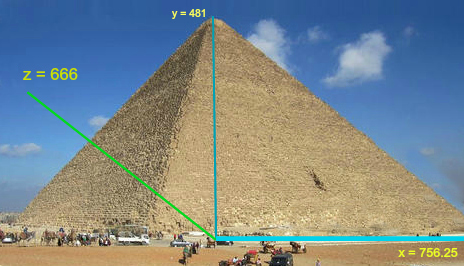said that if the Torah’s first letter, Bet (ב) were 2֯ on a circle, the corresponding remainder of that circle, would be 358, the numerical value of Moshiach, but instead, if it were a percentage of the value of the full 22 Letters (2/1495) then it would be .481… degrees on that circle, aligning it with the height of the Pyramid, 481 ft.

As one enters the Pyramid from a height of 55’ or 2 x 27.5, they pass through a passage 42” wide before encountering the descending passage, which is  26.18 o or φ2 degrees steep, and then they ascend through the grand gallery, sloped at 26o, like the Tetragrammaton(יהוה).  Little known is that when using the average base perimeter as a radius of sphere, we get the volume of a sphere approximately equal to 200,000,000,000,000 or 2.0 x 1014 inches3, a very large precise ball. Maybe it is a hint about spherical time. May just an odd coincidence.

And maybe it too is a coincidence that the height of the Pyramid is 5775”, or (5 x 42 x 27.5) and (52 x 231), as in the 231 Gates of Wisdom described by Abraham Avinu in the Sefer Yetzirah.

Twenty-Two Foundation Letters:

He placed them in a circle

Like a wall with 231 Gates.

The Circle oscillates back and forth.

The word Abraham used for circle can also be translated as sphere. When the 22 letters are arranged in a circle and connected in pairs, 231 bonds are formed. Each arching bond over the top of the sphere is a gate. Completing the circle for each one, completes the sphere and reverses the gate.

The sum of all the letter pairings backwards and forwards of the 231 combinations as specifically found within the words of the Torah total 222,004. Oddly, the sum of the total letter values for those 231 pairs is 31495 or 30,000 + 1495, the numerical value of the 22 letters, with a hint of Pi (314).

And maybe it too is a coincidence that the apothem of the Pyramid is 267 cubits in length and that the base measure of the pyramid divided by 2.6700 = 2018. As we will soon see 2018 was not only a very important time for the world but even more so for spherical time.  Again, cubits and time.

While the apothem of the Pyramid is 267 cubits, the sum of all the integers from 1 to 267 is 35778 as in the 3 5778’s in Pi though 1000. Thus, the higher dimensional value of 267 is 35778.

5778 HC = 2018 CE

Does constructing the Future Holy Temple using the sacred cubit help it to have an interaction with time itself, with the sphere of possibilities and probabilities?

# Intersecting Fields

Of significance, far beyond the Pyramid is that 26φ = 42.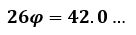This is a relationship that exists in Zeir Anpin. The interaction of 3 major fields. 26 at the level of Binah is the YHVH (יהוה) and 42 is both the 42-Letter Name and the 42 Letters of the Name. Beyond Binah they are something even more significant and beyond our comprehension. Yet their presence is always there and included in the lower iterations of their appearance to us, in the letters, numbers, and their influence over physicality.

The measure of the sacred cubit is a special number. It is the result of the expanded YHVH (יהוה) and of the Phi(φ) field in multiple ways, including the intersection with the 72 field and the Phi(φ) angle in connection with the circle and Pi.

Let us not forget that 72 is also both the aspect of the YHVH (יהוה) at the level of Chochma, and the square of the YHVH (יהוה): (י־יה־יהו־יהוה).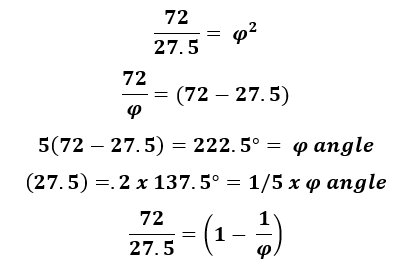From that first letter in the Torah connected to Phi(φ), if we count 304,805/ Phi(φ) letters, or 79975/ Phi(φ) words, or 5845/ Phi(φ) verses we will reach Numbers 1:1, the first chapter in the Book of Numbers and the place in the Torah that divides the Torah into the Phi(φ) proportion in terms of either words, letters, or verses. The 5 Books are split into the first 3 and final 2.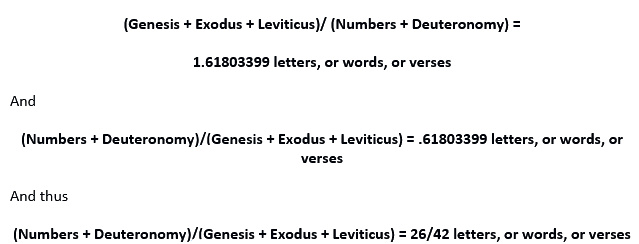Now, if it occurred to you to ask why the 187 chapters of the Torah were not likewise divided into the Phi(φ) proportion when that would seem like a far easier calculation than keeping track of hundreds of thousands of letters ,and words, know this: 187/Phi(φ)  = 115.57236. and 115.57236 jubilee years (Biblical 50-year interval) = 5778.618.

Coincidence? Maybe not, considering the last two portions of the 3rd Book of the Torah, Behar-Bechuchotai, at the end of the first portion of the Phi (.618) split, have 57 and 78 verses respectively, and are most often read together. Moreover, the last portion of the 4th Book contains the recounting of the 42 journeys.

Always five (5): 5 overarching worlds, 5 at the core of the Torah (the 5 Books of Moses), 5 at the core of Phi(φ), 5 at the core of the cubit, 5 at the core of the Alef-bet, and the 5 Essential Elements at the core of Creation.

The Cubit is 27.5” The digits in 27.5 sum to (2 + 7 + 5) = 14, as in the central position of the Essential Cube of Creation, the 5th Essential Element. The digits in 14 also sum (reduce) to 5. The product of the digits in 27.5 is (2 x 7 x 5) = 70, as in the lifespan of King David, of numerical value 14. The gematria cipher P/S in the case of the cubits (27.5”) reveal 70/14 = 5.

The number 5 does not always connote the letter hei (ה) and a gateway but sometimes it does. When the cubit is properly applied you can imagine.

And 42 at the core of the 5 and the entrance to the gate. There is a gate with a revolving sword. There is a sword of 42 letters hidden in the Torah in the 42nd paragraph of the 2nd Book.

# 42 at the Core of it All

When we divide the total value of the 42-Letter name by 506, its first line, we get 3701/506 = 7.31422. This is about one-tenth of 73.1415, which was the result of 9/.123049…, the small gematria value of the 42-Letter Name. It is the numerical small gematria value one of the most key phrases in the Torah, at Exodus 3:14:

I Am That I Am (אהיה־אשר־אהיה)

This bonding of the two higher names of G-d (אהיה), each of numerical value 21, sums to 42. Moreover, because the ordinal value of Ehyeh(אהיה) is also 21, the complete gematria value of each Ehyeh(אהיה) is likewise 42. Furthermore, the ordinal value of asher (אשר), the central word in this key phrase is also 42.

Of the 1024, or precisely 210, different word values in the Torah there are exactly 6 that are found 26 separate times in the Torah—as in the numerical value of the Tetragrammaton(יהוה). The collective value of those 6 words is 3142.

Moreover, the Creator engraved 3142 right into the first 7 letters of the Torah (ברא־שיתב) with their small gematria (2213142).

Even more interesting is that the union of the 2 fields of the 2 most powerful Names, Ehyeh (אהיה) and the Tetragrammaton (יהוה), when expanded into 3 dimensions (iterations) together also equal 3142.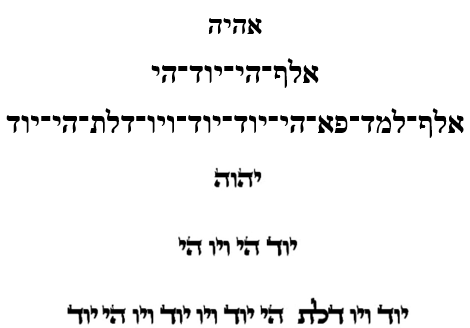The expanded components of Ehyeh (אהיה) are (506 + 110 + 1818) = 2434 and those of the Tetragrammaton (יהוה) are (26 + 72 + 610) = 708, as in the year 5708 when Israel became a nation. Together (2434 + 708) = 3142.

So why 3142? It is obviously illustrative of the fusion of the Pi (314) and 42 fields, but what is behind it?

As we have just seen 3142 represents the fusion of the fields of the 2 higher Names, but when this fusion further interacts with the Phi(φ) field, we get the point of origin of our time bubble: (3142 + 618) = 3760 HC, 0 CE, alternatively it is the event horizon of that same bubble.

Of further note is that 5 letters of Alef(א) from Ehyeh (אהיה) with the YHVH (יהוה) have a numerical value of 27 and a complete value of 54, all representative of the Essential Cube of Creation. When those representative values 54 and 5 are added to the value of the 42-Letter Name, 3701, we also get 3760.

### The Fine-Structure Constant

We have seen both the Phi(φ) and Pi fields entwined in the 14 Triplets of the 42-Letter Name. We have seen how 9 divided by the 42 Letters = 73.141593… or Pi. Now let us examine how the field of the Fine Structure Constant also interacts with the field of 42.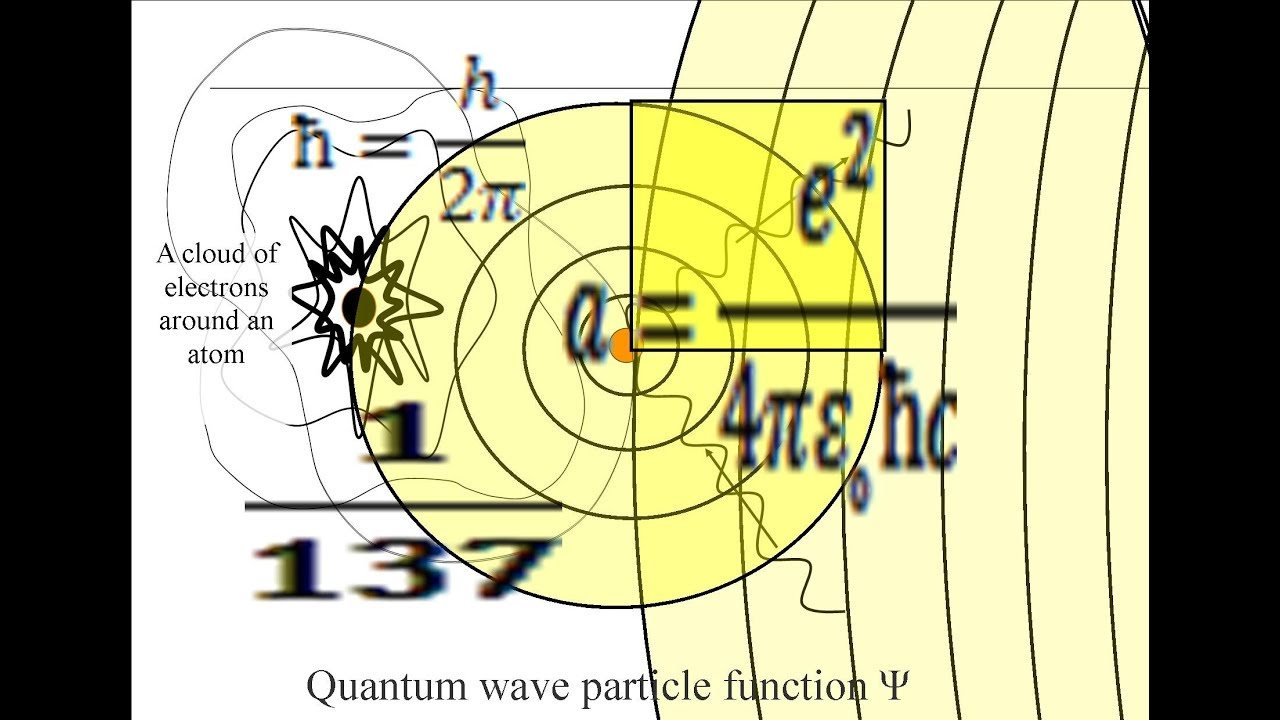When we speak of the interaction between the various fields we are often talking about couplings. Couplings arise naturally in a quantum field theory. A special role is played in relativistic quantum theories by couplings that are dimensionless; i.e., are pure numbers. An example of a dimensionless such constant is the fine-structure constant.

The fine-structure constant α is of dimension 1, meaning it is simply a number and not dependent on other factors, and it is approximately 1/137, apropos since in gematria 137 means both “to receive” and parallel.  More specifically, it is 1/137.035999… It is the “coupling constant” or measure of the strength of the electromagnetic force that governs how we perceive electrically charged elementary particles (e.g., electron, muon) and light (photons) interact.

A more complicated way to see it is as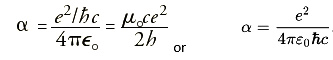where e is the charge of an electron, ℏ = h/2π where h is Planck’s constant, where ε0 = 1/µ0c2 is the electric constant (permittivity of vacuum, also known as free space) and µ0 is the magnetic constant (permeability of vacuum), and where ℏ is the reduced Planck constant and c is the speed of light. This constant is proportional to the square of the coupling strength of the charge of an electron to the electromagnetic field.

Since: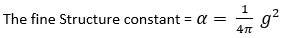The Gauge coupling parameter (g) is: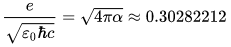There is nothing to calculate here.  Its significance will be apparent momentarily.

Speaking of coupling, we know that the Torah’s first verse of numerical value 2701 and the 42-Letter Name of numerical value 3701 are integrated in multiple ways. What we never pointed out was that the square root of 1370 = 37.01… and the square root of 730 = 27.01…. While (1370 + 730) = 2100 and the total gematria of the Torah is 21009826, and (1370 730) = 640, it is the ties of 730 to the higher dimensional math sum of the integers from 1 – 73 that equal 2701 and the ties of the 1370 to the Fine Structure constant’s 1/137.0… that are more significant.

The Fine Structure constant, 1/137.03599 is equal to .007297353… and while the entire the 2nd line (קרעשטן) of the 42-Letter Name matrix has a numerical value of 729 and the following line permutes to 314 (שדי) and 73 and begins with 53 (נג) as in .007297353, the 2nd Triplet (שטן) of that same the 2nd line has a value of 359 as in  1/137.03599.

Moreover, the small gematria of the 42-Letter Name is 173 and 1/42 x 1/137 = .000173… Yet perhaps most significant of all is that (42 times the Fine Structure constant minus the coupling constant) or (42/αg) = (42/137.03599.302822131) = .0036666… = 11/3000.

Why 11/3000? We do not know for sure, though as previously explained the 42-Letter Matrix is designed to connect with the 11sefirot. Even its first line is the sum of the 11 squares from 12112.  And 3000/11 = 272.72727 or 27 ad infinitim.

The value .0036666 could also be said to equal simply 11/3 and its inverse 3/11 is 273, the factor of physicality in our solar system. The Genesis Torah narrative describes how G-d Created order out of that chaos. Its 9th word (היתה) has a numerical value of 420. That 9th word’s 4 consecutive Torah ordinal values (34+35+36+37) = 142, and its total letter ordinal value is 42 and of course, (420 + 4) for the 4 letters = 424 (Moshiach Ben David).

The 9th word in the Torah with all the ties to 42 usually translates as “was,” as in “and the earth was…” It is juxtaposed to the next 3 words (without form, void, and darkness) of 11 letters in total, and as we just saw 11/3 = 3.66 and 3/11 = 27.3, numbers that greatly define our solar system and physical universe.

It takes 11 words to delineate the first 42 letters of the Torah. The sum of their Torah ordinal values is 1 – 42 = 903, yet the breakdown of each word has meaning. Interestingly, the square root of the Torah ordinal values (41 + 42) of the two-letters that pertain to the 42 from the 11th word is 9.110

When we examine the words pertaining to the time before the disorder, when the order happened naturally and G-d did not have to actively intervene with numerous modalities of separation, a time before a firmament was set in the heavens to separate the two existences, we find a certain type of result. The sum of the Torah’s ordinal values for the first word is (1+2+3+4+5+6) = 21, which as we previously stated is the same as Ehyeh(אהיה), The Name of G-d associated with the hyperspace (Binah) upper-level dimension. The sum for the first two words is 45, representing Adam and the aspect of the Tetragrammaton connected to that “in between” or processing level, Zeir Anpin. While the 2nd and 3rd words together equal 84, The 3rd and 4th words together equal 91, with its connection to 13, the unification of the Names, etc. The Torah’s ordinal values for first word through the 5th word sum to 1 – 21 = 231, as in the 231 Gates of Wisdom just discussed. Nevertheless, the sums for the 4th and 5th words together equal 126, as in the face of each side of the Essential Cube of Creation, which also means that the sum for the 4 words of the 2nd – 5th must sum to 210, the height of the pyramid, 1 – 20, etc. Then while the 1st through 6th equal 300 with its connection to various Names of G-d including Elohim, Adonai and the Tetragrammaton as previously explained, the 6th, 7th and 8th total collectively 330, the complementary base of the Pyramid.  The 1st through 9th words all together equal 703, as in 19 x 37, and the value of the Torah’s 6th and 7th words V’et H’ertz (26.0% of first verse), while the first ten (10) all together equal 820, the value of the core verse of the Torah, “Love they neighbor as thyself.”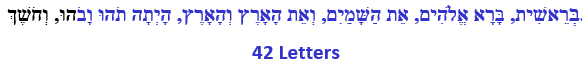As you may have noticed, the Torah’s ordinal values for the first word = 42/2; the 2nd and 3rd = 42 x 2; the 4th and 5th = 42 x 3 and the 2nd – 5th = 42 x 5, making the first 5 words = (42 x 5.5). Genesis 1:1 and 5.5/5 = 1.1.   Always 5 at the core and 42 at the core of 5.

The spherical schematic of time will morph a bit as we unfold it, one concept at a time, as have the concepts encoded into the Torah and our universe. For the moment it is important to understand it as the interaction of the Phi(φ) and Pi fields that create the sphere of time, generating infinite pathways for the point of origin. We still have to delve into why it is a bubble, at least locally, and not infinite. Each moment in time is a concentric ring of potentiality emanating from that point of origin. Why is there an event horizon?

You probably never thought about this, but the Torah has a start date (0 HC) and an end date, 2488 HC, 1273 BCE in the Western Calendar. While 2488 would seem to hint at the 248 dimensions of the E8 Lattice, 1273 is the Universal Harmonic that squares our circles and our universe, defines the structure of our solar system, and inspires Bill Gates (Mrna-1273) to vaccinate every last person on the planet before the end of this year. For anyone that has not been following this series from its inception, you can find the entire work here.